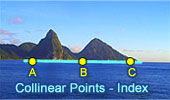# Collinear Points, Theorems and Problems - Table of Content 4Geometry Problem 824
Desargues' Theorem, Triangles in Perspective.

Geometry Problem 723.
Squares, Circumscribed Circles, Collinear points.

Geometry Problem 722.
Squares, Circumscribed Circles, Collinear points.

Geometry Problem 721.
Triangle, Orthocenter, Circumcircle, Altitude, Collinear points.

Geometry Problem 710.
Parallelogram, Midpoint, Diagonal, Collinear Points, Metric Relations, Triangle, Similarity, Congruence.

Go to page:  Previous | 1 | 2 | 3 | 4 | 5 | 6 | 7 | 8 | 9 | 10 | Next